Tamilnadu State Board New Syllabus Samacheer Kalvi 11th Maths Guide Pdf Chapter 2 Basic Algebra Ex 2.2 Text Book Back Questions and Answers, Notes.

## Tamilnadu Samacheer Kalvi 11th Maths Solutions Chapter 2 Basic Algebra Ex 2.2

Question 1.
Solve for x
(i) |3 – x| < 7
-7 < 3 – x < 7 3 – x > -7
-x > -7 -3 (= -10)
-x > -10 ⇒ x < 10
3 – x < 7
– x < 7 – 3 (= 4)
– x < 4x > -4 … .(2)
From (1) and (2)
⇒ x > -4 and x < 10
⇒ -4 < x < 10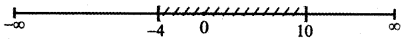(ii) |4x – 5| ≥ – 2
|4x – 5| ≥ -2
(4x – 5) ≤ -(-2) or (4x – 5) ≥ -2
(4x – 5) ≤ 2 or (4x – 5) ≥ -2
4x ≤ 2 + 5 or 4x ≥ -2 + 5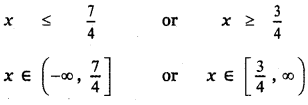∴ x ∈ (-∞, ∞) = R

(iii) |3 – $$\frac{3}{4}$$x| ≤ $$\frac{1}{4}$$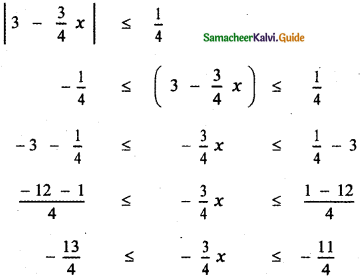Multiplying by 4, we have
– 13 ≤ – 3x ≤ – 11 ——– (1)
We know that a < b ⇒ $$\frac{\mathrm{a}}{\mathrm{y}}$$ > $$\frac{\mathrm{b}}{\mathrm{y}}$$ when y < 0
Divide equation (1) by – 3, we have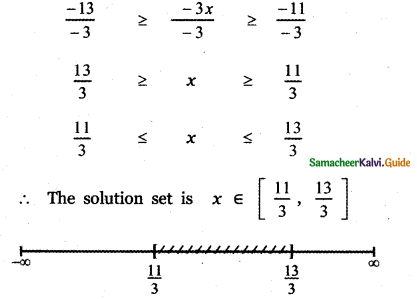(iv) |x| – 10 < – 3
|x| – 10 < – 3
|x| < – 3 + 10
|x| < 7
– 7 < x < 7
∴ The solution set is (-7, 7)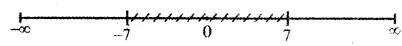Question 2.
Solve $$\frac{1}{|2 x-1|}$$ < 6 and express the solution using the interval notation.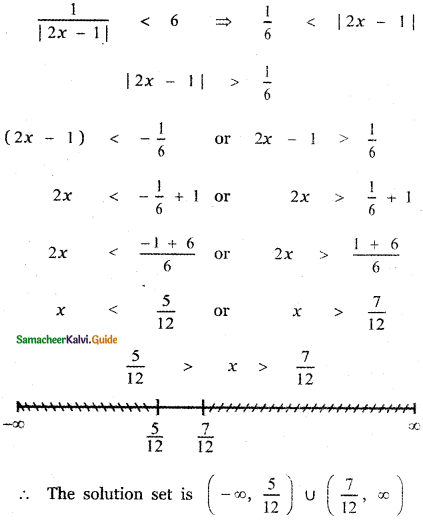Question 3.
Solve – 3 |x| + 5 ≤ – 2 and graph the solution set in a number line.
-3|x| + 5 ≤ – 2
⇒ -3 |x| ≤ – 2 – 5 (= -7)
-3|x| ≤ – 7 ⇒ 3 |x| ≥ 7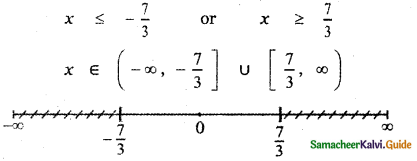Question 4.
SoIve 2|x + 1| – 6 ≤ 7 and graph the solution set in a number line.
Given 2|x + 1| – 6 ≤ 7
2|x + 1| ≤ 7 + 6
2|x + 1| ≤ 13
|x + 1| ≤ $$\frac{13}{2}$$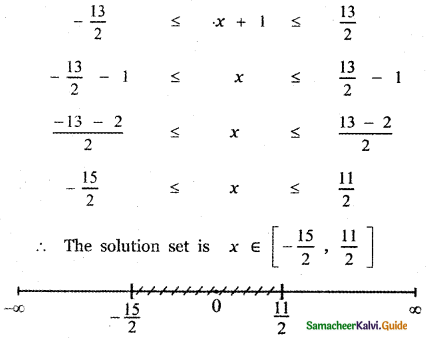Question 5.
Solve $$\frac{1}{5}$$ |10x – 2| < 1
Given $$\frac{1}{5}$$ |10x – 2| < 1
|10x – 2| < 5
-5 < (10x – 2) < 5
– 5 + 2 < 10x < 5 + 2
– 3 < 10x < 7
$$-\frac{3}{10}$$ < x < $$\frac{7}{10}$$
∴ The solution set is x ∈ $$\left(-\frac{3}{10}, \frac{7}{10}\right)$$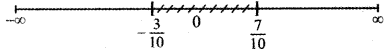Question 6.
Solve |5x – 12| < – 2